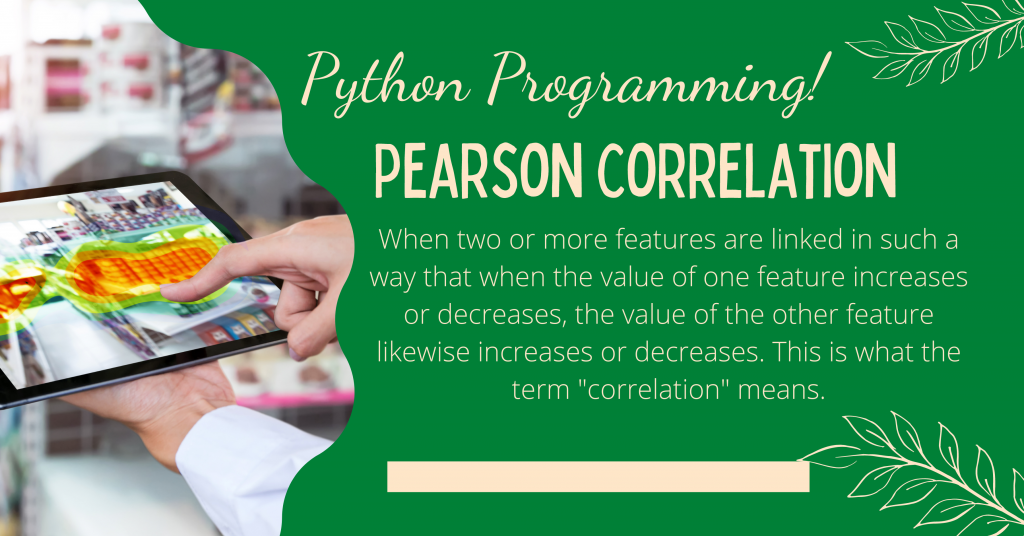# Pearson Correlation – Implementing Pearson Correlation in PythonIn this tutorial, I’ll guide you through the Python implementation of Pearson Correlation. When two or more features are linked in such a way that when the value of one feature increases or decreases, the value of the other feature likewise increases or decreases. This is what the term “correlation” means.

## Introduction to Correlation

Finding the link between variables is what correlation is all about. In data science, we utilize correlation to discover characteristics that are favorably and negatively associated with one another so that we may train a machine learning model using the best features.

The degree of correlation ranges from -1 to 1.

1. When the correlation between the characteristics is 1, the features are positively associated with each other.
2. When the correlation between the features is -1, the features are negatively linked with each other.
3. When the correlation between the characteristics equals zero, we may conclude that there is no association between the features.

## Introduction to Pearson Correlation

Pearson correlation is a statistical approach for determining the strength of a linear relationship between two or more features.

One of the best examples of Pearson’s correlation is demand and supply. For example, when the demand for a product grows, the supply of that product increases, and when the demand for that product decreases, the supply of that product decreases. There is a positive correlation between demand and supply of a product.

## Implementation of Pearson Correlation in Python

In order to observe the correlation, we need to follow a number of steps which are described below.

The first step in any program is loading the necessary modules (if needed). For this program, we would be required to import the `pandas` module. We would, later on, load the dataset using the `read_csv` function. You can find the dataset here.

```import pandas as pd
```

### Step 2 – Finding Correlation between all the features

In order to find the correlation, we will make use of the `corr` function and pass the `method` as `pearson` as we aim to find the Pearson Correlation among features.

```movies['Rotten Tomatoes'] = movies["Rotten Tomatoes"].str.replace("%", "").astype(float)
movies.drop("Type", inplace=True, axis=1)
correlations = movies.corr(method='pearson')
```

### Step 3 – Visualizing the Correlation

In order to visualize the correlation, we will make use of the `seaborn` plot and import the `seaborn` and the `matplotlib` modules. Finally, we make use of the `heatmap` function and pass the correlation we created in the previous step.

```import seaborn as sns
import matplotlib.pyplot as plt
sns.heatmap(correlations)
plt.show()
```